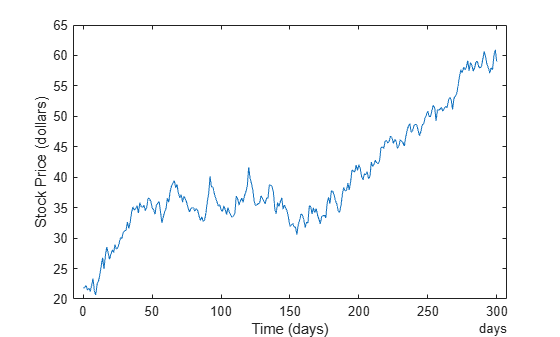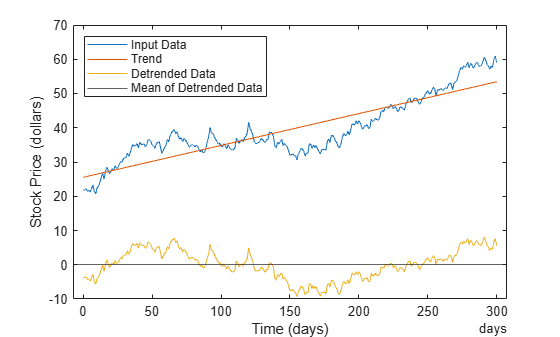# Remove Linear Trends from Timetable Data

This example shows how to remove a linear trend from daily closing stock prices in a timetable to emphasize the price fluctuations about the overall increase. If the data does have a trend, detrending it forces its mean to zero and reduces overall variation. The example simulates stock price fluctuations using a distribution taken from the `gallery` function.

Create a simulated timetable containing the daily price of a stock.

```t = 0:300; D = days(t); dailyFluct = randn(size(t)); price = cumsum(dailyFluct) + 20 + t/100; tt = timetable(D',price',VariableNames="StockPrice");```

Find the average daily price of a stock.

`mean(tt.StockPrice)`
```ans = 28.2260 ```

Plot and label the data. Notice the systematic increase in the stock prices.

```plot(tt,"Time","StockPrice"); legend("Original Data","Location","northwest"); xlabel("Time (days)"); ylabel("Stock Price (dollars)");```Apply `detrend`, which performs a linear fit to the stock prices, removes the trend, and appends the detrended data to the input timetable.

`tt = detrend(tt,ReplaceValues=false);`

Compute the trend line by subtracting the detrended data from the input data.

```trend = tt.StockPrice - tt.StockPrice_detrended; tt = addvars(tt,trend,NewVariableNames="Trend")```
```tt=301×3 timetable Time StockPrice StockPrice_detrended Trend _______ __________ ____________________ ______ 0 days 20.538 -14.588 35.126 1 day 22.382 -12.698 35.08 2 days 20.133 -14.901 35.034 3 days 21.005 -13.983 34.988 4 days 21.334 -13.608 34.942 5 days 20.036 -14.86 34.896 6 days 19.612 -15.238 34.85 7 days 19.965 -14.839 34.804 8 days 23.553 -11.204 34.758 9 days 26.333 -8.3791 34.712 10 days 24.993 -9.6729 34.666 11 days 28.038 -6.582 34.62 12 days 28.773 -5.8006 34.574 13 days 28.72 -5.8077 34.528 14 days 29.445 -5.0369 34.482 15 days 29.25 -5.1859 34.436 ⋮ ```

Find the average of the detrended data.

`mean(tt.Trend)`
```ans = 28.2260 ```

As expected, the detrended data has a mean very close to 0.

Display the results by adding the trend line, the detrended data, and its mean to the graph.

```plot(tt,"StockPrice") hold on plot(tt,"Trend") plot(tt,"StockPrice_detrended") plot(tt.Time,zeros(size(t))) legend("Original Data","Trend","Detrended Data",... "Mean of Detrended Data","Location","northwest") xlabel("Time (days)"); ylabel("Stock Price (dollars)");```